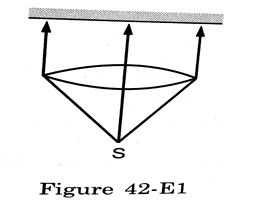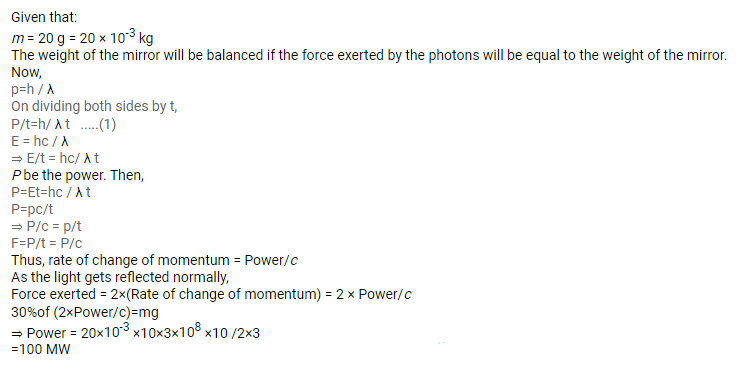# A totally reflecting, small plane mirror placed horizontally faces a parallel beam of light,

Question:

A totally reflecting, small plane mirror placed horizontally faces a parallel beam of light, as shown in the figure. The mass of the mirror is $20 \mathrm{~g}$. Assume that there is no absorption in the lens and that $30 \%$ of the light emitted by the source goes through the lens. Find the power of the source needed to support the weight of the mirror.

Figure 42-E1Solution: